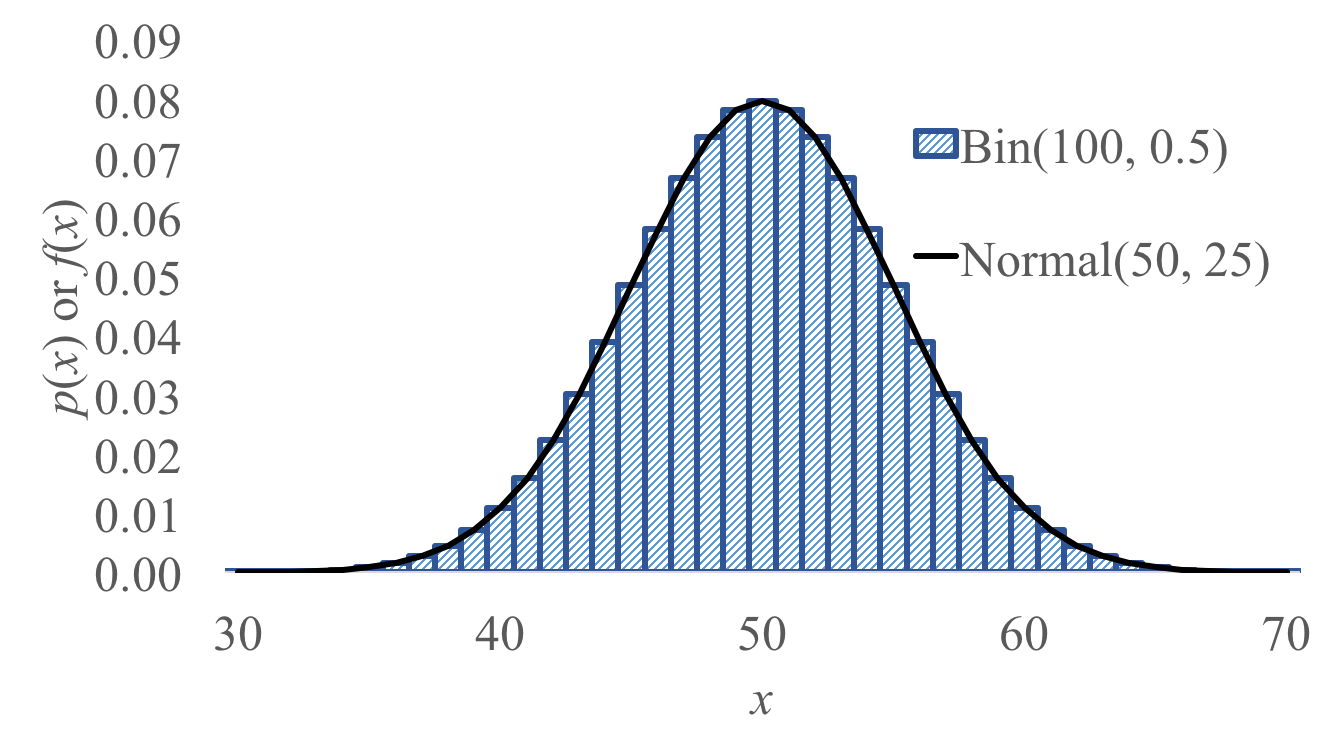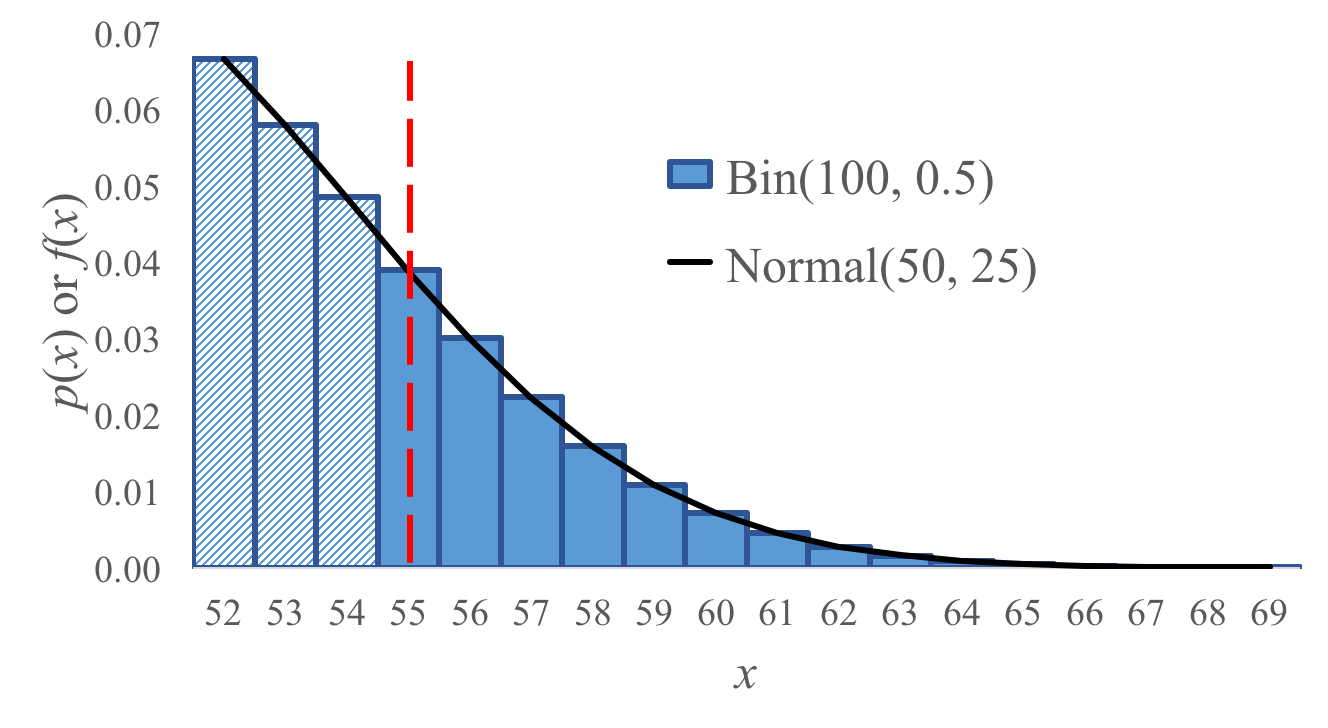# Binomial Approximation

There are times when it is exceptionally hard to numerically calculate probabilities for a binomial distribution, especially when $n$ is large. For example, say $X \sim \text{Bin}(n = 10000, p = 0.5)$ and you want to calculate $\p(X > 5500)$. The correct formula is: \begin{align} \p(X > 55) &= \sum_{i = 5500}^{10000} \p(X=x) \\ &= \sum_{i = 5500}^{10000}{10000 \choose i}p^i(1-p)^{10000-i} \end{align}

That is a difficult value to calculate. Luckily there is an easier way. For deep reasons which we will cover in our section on "uncertainty theory" it turns out that a binomial distribution can be very well approximated by both Normal distributions and Poisson distributions if $n$ is large enough.

Use the Poisson approximation when $n$ is large (>20) and $p$ is small (<0.05). A slight dependence between results of each experiment is ok

Use the Normal approximation when $n$ is large (>20), and $p$ is mid-ranged. Specifically it's considered an accurate approximation when the variance is greater then 10, in other words: $np(1-p)>10$. There are situations where either a Poisson or a Normal can be used to approximate a Binomial. In that situation go with the Normal!

## Poisson Approximation

When defining the Poisson we proved that a Binomial in the limit as $n \rightarrow \infty$ and $p = \lambda/n$ is a Poisson. That same logic can be used to show that a Poisson is a great approximation for a Binomial when the Binomial has extreme values of $n$ and $p$. A Poisson random variable approximates Binomial where $n$ is large, $p$ is small, and $\lambda = np$ is “moderate”. Interestingly, to calculate the things we care about (PMF, expectation, variance) we no longer need to know $n$ and $p$. We only need to provide $\lambda$ which we call the rate. When approximating a Poisson with a Binomial, always choose $\lambda = n \cdot p$.

There are different interpretations of "moderate". The accepted ranges are $n > 20$ and $p < 0.05$ or $n > 100$ and $p < 0.1$.

Let's say you want to send a bit string of length $n = 10^4$ where each bit is independently corrupted with $p = 10^{-6}$. What is the probability that the message will arrive uncorrupted? You can solve this using a Poisson with $\lambda = np = 10^4 10^{-6} = 0.01$. Semantically, $\lambda = 0.01$ means that we expect $0.01$ corrupt bits per string, assuming bits are continuous. Let $X \sim Poi(0.01)$ be the number of corrupted bits. Using the PMF for Poisson: \begin{align*} P(X = 0) &= \frac{\lambda^i}{i!}e^{-\lambda}\\ &= \frac{0.01^0}{0!}e^{-0.01}\\ &\sim 0.9900498 \end{align*} We could have also modelled X as a binomial such that $X \sim Bin(10^4, 10^{-6})$. That would have been impossible to calculate on a computer but would have resulted in the same number (up to the millionth decimal).

## Normal Approximation

For a Binomial where $n$ is large and $p$ is mid-ranged, a Normal can be used to approximate the Binomial. Let's take a side by side view of a normal and a binomial:Lets say our binomial is a random variable $X \sim \text{Bin}(100, 0.5)$ and we want to calculate $P(X \geq 55)$. We could cheat by using the closest fit normal (in this case $Y \sim N(50, 25)$). How did we choose that particular Normal? Simply select one with a mean and variance that matches the Binomial expectation and variance. The binomial expectation is $np = 100 \cdot 0.5 = 50$. The Binomial variance is $np(1-p) = 100 \cdot 0.5 \cdot 0.5 = 25$.

You can use a Normal distribution to approximate a Binomial $X\sim \Bin(n,p)$. To do so define a normal $Y \sim (E[X], Var(X))$. Using the Binomial formulas for expectation and variance, $Y \sim (np, np(1-p))$. This approximation holds for large $n$ and moderate $p$. That gets you very close. However since a Normal is continuous and Binomial is discrete we have to use a continuity correction to discretize the Normal.\begin{align*} P(X = k) \sim P\left(k - \frac{1}{2} < Y < k + \frac{1}{2}\right) = \Phi\left( \frac{k - np + 0.5}{\sqrt{np(1-p)}} \right) - \Phi\left( \frac{k - np - 0.5}{\sqrt{np(1-p)}} \right) \end{align*} You should get comfortable deciding what continuity correction to use. Here are a few examples of discrete probability questions and the continuity correction: \begin{align*} &\text{Discrete (Binomial) probability question} && \text{Equivalent continuous probability question}\\ &P(X=6) && P(5.5 < X < 6.5) \\ &P(X\geq6) && P(X > 5.5) \\ &P(X > 6) && P(X > 6.5) \\ &P(X < 6) && P(X < 5.5) \\ &P(X \leq 6) && P(X < 6.5) \\ \end{align*}

Example: 100 visitors to your website are given a new design. Let $X$ = # of people who were given the new design and spend more time on your website. Your CEO will endorse the new design if $X \geq 65$. What is $P(\text{CEO endorses change}|\text{it has no effect})$?

$E[X] = np = 50$. $\Var(X) = np(1-p) = 25$. $\sigma = \sqrt{\Var(X)} = 5$. We can thus use a Normal approximation: $Y \sim \mathcal{N}(\mu = 50, \sigma^2 = 25)$. \begin{align*} P(X \geq 65) &\approx P(Y > 64.5) = P\left(\frac{Y - 50}{5} > \frac{64.5 - 50}{5}\right) = 1 - \Phi(2.9) = 0.0019 \end{align*}

Example: Stanford accepts 2480 students and each student has a 68% chance of attending. Let $X$ = # students who will attend. $X\sim \Bin(2480, 0.68)$. What is $P(X > 1745)$?

$E[X] = np = 1686.4$. $\var(X) = np(1-p) = 539.7$. $\sigma = \sqrt{\Var(X)} = 23.23$. We can thus use a Normal approximation: $Y \sim \mathcal{N}(\mu = 1686.4, \sigma^2 = 539.7)$. \begin{align*} P(X > 1745) &\approx P(Y > 1745.5) \\ &\approx P\left(\frac{Y - 1686.4}{23.23} > \frac{1745.5 - 1686.4}{23.23}\right) \\ &\approx 1 - \Phi(2.54) = 0.0055 \end{align*}